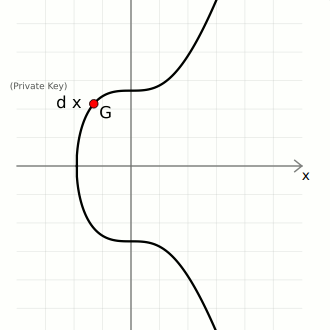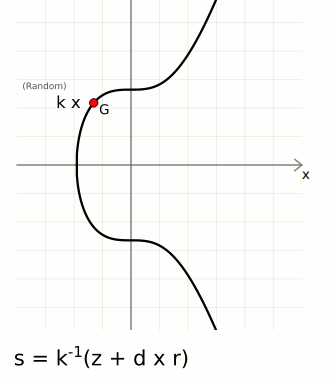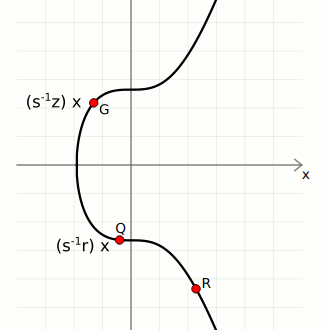# Key generation, Signing and Verifying transactions in Bitcoin

Get FREE domain for 1st year and build your brand new siteIn this article, we learn how the ECDSA is used in the signing and verification of transactions in Bitcoin.

1. Introduction.
2. Generating Keys.
3. Signing a Transaction.
4. Verifying a Transaction.
5. Summary.
6. References.

### Prerequisites

ECDSA Algorithm and Elliptic Curves.

## Introduction

In the previous article, we learned about the Elliptic curve and the mathematical concepts involved.

The Elliptic Curve finds applications in public-key cryptography, specifically a cryptographic algorithm referred to as the Elliptic Curve Digitial Signature Algorithm.

In this article, using python we will implement three functions, the first for generating the key pair, the second for signing, and the final function for verifying a signature.

## Generating Keys.

Public-key cryptography also known as asymmetric cryptography is at the heart of blockchain networks. Bitcoin uses ECDSA in generating key pairs, signing, and verification.

Consider the following elliptic curve;d is the private key, a randomly generated number within a specific range. G the generator point and Q the public key, which is obtained by multiplying the generator point G by the random number d, i.e, dG = Q.

#### Trapdoor function.

This concept means that even if we share the public key with the public and there will be no way of coming up with its key pair, the private key. The only way to calculate the private key would be to multiply the generator point G by different numbers until the public key matches.

This is a brute force approach and it is very slow and resource-intensive. Thus, elliptic curve multiplication is referred to as a trap door function since it is easy to go one way but difficult to reverse. This is what asymmetric cryptography is based on.

In conclusion, a one-way mathematical function connects the key pair meaning we can use each independent of the other to calculate points on the curve, which we will use for digital signatures.

## Signing a Transaction.

Three components are needed for the signing of a message or transaction;

• Random number, this is used for randomness, a key aspect of digital signature's security.
• Message Hash, the message has been passed through a hashing function into a fixed-size string, the hashing function implemented is SHA-256.
• Private key*, this is to be kept private, it is the source of the public key.

A signature has two components;

• A random point on the curve, to obtain this number we choose a random number k and multiply it to the generator point to get the random point R. We use the x-coordinate of this point - r.
• A number to accompany the random point*, this is a unique number generated from combining message hash z and private key d that is bound to the random point using r.We have;
R = kG
r = ${\mathrm{R}}_{\mathrm{x}}$ mod n
s = ${\mathrm{k}}^{-1}$(z + dr) mod n

Above -1 is used to perform a modular inverse of the number. The digital signature consists of the value r and s.

s the unique value is used as a way of randomly generating point r. Given r and s starting from the generator point G, a person can use s to get a random point r. In this case, only a person whose private key is valid can get the valid path to random point r provided by s.

The following demonstrates this using python code;

``````def sign_message(private_key, message):
z = hash_message(message)

r = 0
s = 0

while not r or not s:
k = random.randrange(1, curve.n)
x, y = multiply(k, curve.g)

r = x % curve.n
s = ((z + r * private_key) * mod_inv(k, curve.n)) % curve.n

return (r, s)
``````

## Verifying a Transaction.

For us to be able to verify a message, three key pieces are important;

• The public key, is publicly shared. It belongs to the creator of the digital signature.
• The message, This is the data signed by the sender.
• The digital signature, is created for the message, the creator also owns the private key for the public key used to encrypt the message.

Given these three pieces of information, we can calculate two points on the curve;

• First, the generator point G, then multiply it by the inverse(s) * z
• Second, the public point Q, then multiply it by the inverse(s) * r
• Finally we add these two points to get point three shown below;That is;
(${\mathrm{s}}^{-1}$z)G + (${\mathrm{s}}^{-1}$r)Q = R

If the final point matches with the random point, the signature is considered valid, otherwise, it is invalid.

For any encrypted message, its digital signature was created by the owner of the private key that the public key was created from. This means, s is unobtainable since it can be used with the public key Q to reach random point R.

This also maintains immutability whereby, message contents cannot be changed since the final point will be different meaning the verification fails.

The following concept is implemented in python code below;

``````def verify_signature(public_key, message, signature):
z = hash_message(message)

r, s = signature

w = mod_inv(s, curve.n)
u1 = (z * w) % curve.n
u2 = (r * w) % curve.n

multiply(u2, public_key))

if (r % curve.n) == (x % curve.n):
return '### Matched Signature ###'
else:
return '### Invalid Signature ###'
``````

## Summary

A nonce is a number only used once. Randomization is important to avoid generating similar digital signatures and also to keep anyone from being able to generate the private key.

Multiplication using the modular multiplicative inverse is the same thing as division in elliptic curve mathematics.

With this article at OpenGenus, you must have the complete idea of Key generation, Signing and Verifying transactions in Bitcoin.

Full code